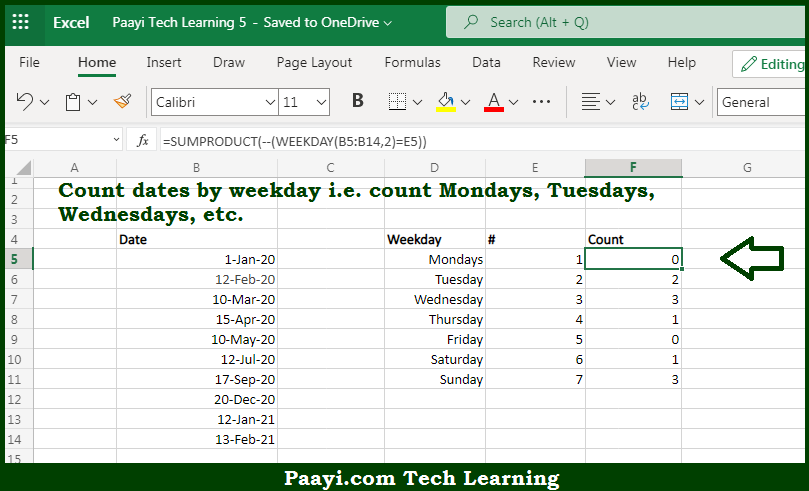# Learn How to Count Cells By Dates of Week in Microsoft Excel

Written by | 0 Comments | 624 Views

In this article, you will learn how to COUNT various things in Microsoft Excel using a single or combination of functions and its purpose in Microsoft Excel. You will also get to know how to count cells by dates of week and see the generic formula

Count Cells By Dates of Week in Microsoft Excel

The main purpose of this formula is to count cells by dates of every day of the week, like Monday to Sunday. Here we will learn how to count the range of cells by dates of the week in Microsoft Excel. That implies, with the help of a formula based on the SUMPRODUCT and WEEKDAY functions you can able to count the count dates of every day of the week. So, with the help of this formula, you can able to count the range of cells by dates of the week.

General Formula to COUNT Cells By dates of Week

=SUMPRODUCT(--(WEEKDAY(dates)=day_num))

The Explanation for the COUNT Cells By Dates of WeekSo we know that with the help of the given formula above you can able to count dates of every day of the week, like Monday to Sunday. Here we will learn how to count the range of cells by dates of the week in Microsoft Excel. As we know that, the COUNTIF function is used to count the number of cells in the range. And you must be thinking why we have not used COUNTIF or COUNTIFS functions? As can be a good solution, however, without adding a helper column that contains a weekday value, there is no way to create criteria for COUNTIF to count weekdays in a range of dates.

That's why using the versatile SUMPRODUCT function, we can able to handle arrays properly without the need to use Control + Shift + Enter. So, with the help of this formula, you can able to count cells by dates of every day of the week.